Study P3 Mathematics Parallel and Perpendicular Lines - Geniebook# Parallel And Perpendicular Lines

1. Understanding and identifying Perpendicular Lines
2. Drawing perpendicular lines using a ruler and(or) a set square on a square grid
3. Drawing perpendicular lines using a ruler and(or) a set square without a grid
4. Understanding and identifying Parallel Lines
5. Drawing parallel lines using a ruler and(or) a set square
6. Drawing parallel lines using a ruler and(or) a set square without a grid

## 1. Understanding And Identifying Perpendicular Lines

Perpendicular lines are straight lines that meet at a right angle.The corner of a ruler is a right angle. We can use it to check for right angles.ST is perpendicular to UV.

We can write it as ST $$\perp$$ UV where stands for is perpendicular to.

Question 1:

Look at the diagram below.There are __________ pair(s) of perpendicular lines.

Solution:AB $$\perp$$ GH

CD $$\perp$$ EF

There are 2 pairs of perpendicular lines.

2

Question 2:

Look at the diagram below.Which line is perpendicular to AB?

1. CD
2. EF
3. GH
4. LK

Solution:EF $$\perp$$ AB because EF and AB intersect each other at a right angle.

(2) EF

Question 3:

Look at the diagram below.Which two lines are perpendicular to each other?

1. FB and GC
2. FB and DA
3. HJ and GC
4. GC and IE

Solution:(3) HJ and GC

Question 4:

Look at the diagram below.Which two lines are perpendicular to each other?

1. AB and BC
2. BC and CD
3. DE and EF
4. EF and FG

Solution:(4) EF and FG

Question 5:

Look at the figure below.Which line is perpendicular to AB?

1. CD
2. EF
3. GH
4. IJ

Solution:

Lines which meet at a right angle are called perpendicular lines.Hence, AB $$\perp$$ CD.

(1) CD

Question 6:

Look at the figure below.Which line is perpendicular to AD?

1. AE
2. ED
3. AB
4. EF

Solution:(2) ED

Question 7:

Look at the figure below.How many pair(s) of perpendicular lines are there in the figure?

1. 1
2. 2
3. 3
4. 4

Solution:

Identify the number of right angles in the figure.

There are 4 right angles in the figure.

There are 4 pairs of perpendicular lines.AB $$\perp$$ AJ,  AJ $$\perp$$ JI,  GF $$\perp$$ FE  and  FE $$\perp$$ ED.

(4) 4

## 2. Constructing Perpendicular Lines On A Square Grid

Using a ruler and a set-square, a line that is perpendicular to the given line can be drawn on a square grid in the following way.## 3. Constructing Perpendicular Lines Without A Grid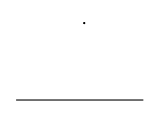We can construct a line that is perpendicular to the given line without a grid using a set square.

Align the base of the set square with the line and draw a straight line through the dot as shown in the picture.Question 1:

Draw a line passing through the point that is perpendicular to the given line.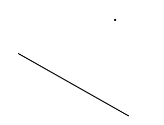Solution:## 4. Understanding And Identifying Parallel Lines

Parallel lines are lines that do not intersect at any point.How to identify whether 2 lines are parallel?

1. They do not meet or touch each other when extended in both directions.
2. The distance between the two lines must always be the same.

Question 1:

Look at the diagram below.There are __________ pair(s) of parallel lines.

Solution:CD is parallel to EF.

JI is parallel to LK.

There are 2 pairs of parallel lines.

2

Question 2:

Look at the diagram below.Which line is parallel to GH?

1. AB
2. CD
3. EF
4. LK

Solution:(2) CD

Question 3:

Look at the diagram below.Which two lines are parallel to each other?

1. AB and CD
2. AB and GH
3. IJ and EF
4. CD and IJ

Solution:(4) CD and IJ

Question 4:

Look at the diagram below.Which two lines are parallel to each other?

1. AG and CD
2. BC and DE
3. AB and DE
4. FG and EF

Solution:

AG and CD are parallel to each other as the distance apart from each other is the same and if extended, they will not intersect.(1) AG and CD

Question 5:

Look at the figures below.Which of the following shows a pair of parallel lines?

1. A
2. B
3. C
4. D

Solution:(1) A

Question 6:

Look at the figure below.Which of the following is parallel to CD?

1. ED
2. AG
3. HI
4. BC

SolutionAG, HF and IE are parallel to CD.

(2) AG

Question 7:

Look at the figure below.How many pair(s) of parallel lines are there?

1. 1
2. 2
3. 3
4. 4

SolutionAB is parallel to CD.

This is a parallelogram.

There are 2 pairs of parallel lines.

(2) 2

## 5. Constructing Parallel Lines On A Square Grid

Using a ruler, draw a line with equal number of squares apart throughout the line.Question 1:

Draw a line that is parallel to the given line.Solution:## 6. Constructing Parallel Lines Without A Grid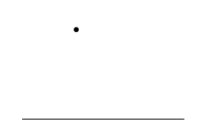We can construct a line that is parallel to the given line without a grid using a set square and a ruler.

Align the set square with the given line.

Place a ruler along the base of the set square.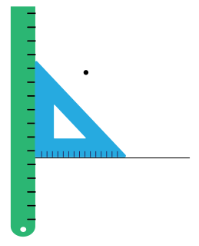Slide the set square along the ruler until it meets the given point.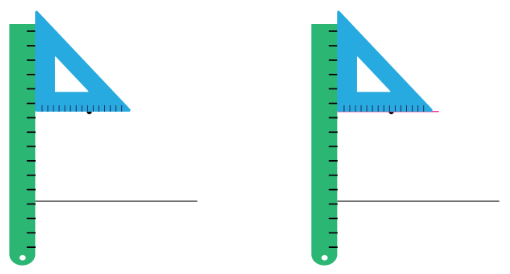Draw a line across.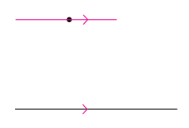Question 1:

Draw a line passing through the point that is parallel to the given line.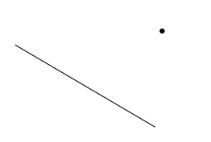Solution:

Align the set square with the given line.

Place a ruler along the base of the set square.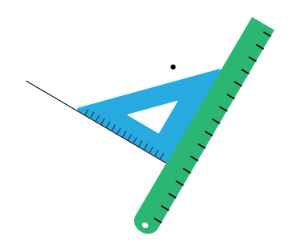Slide the set square along the ruler until it meets the given point.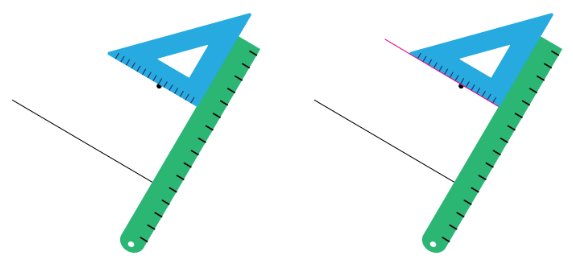Draw a line passing through the given point.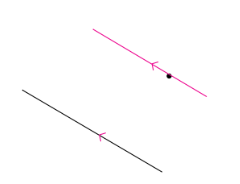Continue Learning
Volume Money
Mass Fractions
Addition And Subtraction Whole Numbers 1
Whole Numbers 3 Whole Numbers 6
Bar Graphs Angles
Multiplication And Division Length, Mass And Volume
Length Area And Perimeter
Parallel And Perpendicular LinesPrimaryPrimary 1Primary 2Primary 3EnglishMaths
Volume
Money
Mass
Fractions
Whole Numbers 1
Whole Numbers 3
Whole Numbers 6
Bar Graphs
Angles
Multiplication and Division
Length, Mass And Volume
Length
Area and Perimeter
Parallel And Perpendicular LinesSciencePrimary 4Primary 5Primary 6SecondarySecondary 1Secondary 2Secondary 3Secondary 4